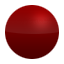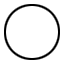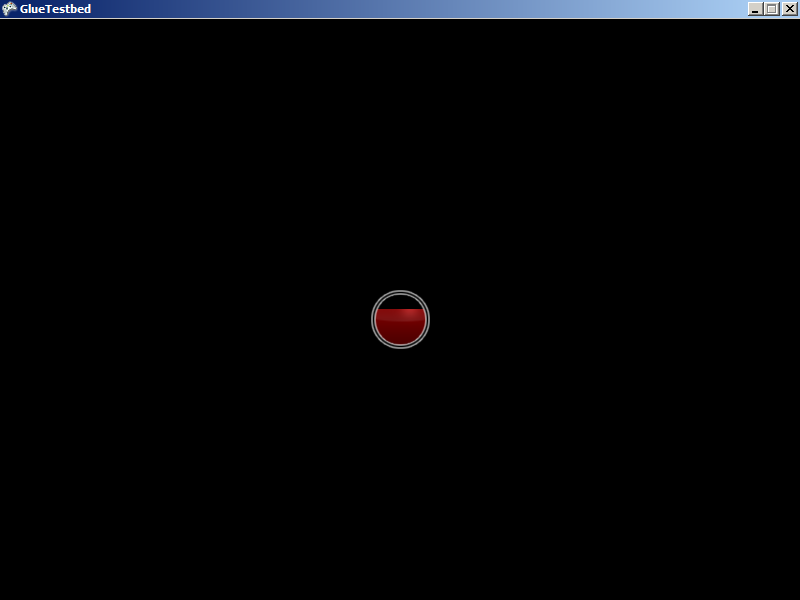# BottomTextureCoordinate

## Introduction

The TopTextureCoordinate, BottomTextureCoodinate, LeftTextureCoordinate, and RightTextureCoordinate properties control the coordinates in “uv space” of the top, bottom, left, and right sides of the Sprite. In other words, these values can be used to make a Sprite draw part of a texture instead of the entire thing. These properties are often used when working on games that use sprite sheets.

The default values are as follows:

• TopTextureCoordinate = 0
• BottomTextureCoordinate = 1
• LeftTextureCoordinate = 0
• RightTextureCoordinate = 1

## What are “texture coordinates” and how do they compare to pixels

Texture coordinates are often used in games (not just FlatRedBall) to measure points on a texture. Texture coordinates are often displayed as two values separated by commas within parenthesis. For example:

```(0, 0)
```

and

```(.75, .25)
```

The texture coordinate is a coordinate which measures location on a texture, but this coordinate does not use pixels. For example, if you’re used to using pixels, you may be familiar with (0,0) being the top-left of an image. If your image is 32 pixels tall and 32 pixels wide, then the bottom-right of the image would be (32, 32).

In texture coordinates, the top left is (0, 0). The bottom-right is (1, 1). You can think of texture coordinates as representing a percentage. In other words, a value of 0 means 0%. 1 means 100%. A value of 0.5 means 50%. Therefore, a value of (.5, 0) means “50% of the width (starting at the left) and 0% of the height (starting at the top). Therefore, the very center of the image is (.5, .5).

## Code example

Texture coordinates can be adjusted to create objects which show only part of a texture, such as health bars. The following code shows how to create a Diablo-like health meter (of course, using a red ball):

Files used:

• Media:Fill.png• Media:Frame.pngAdd the following at class scope:

```Sprite mFrameSprite;
Sprite mFillSprite;
float mFillRatio = 1;
```

Add the following to Initialize after initializing FlatRedBall:

```SpriteManager.Camera.UsePixelCoordinates(false);

// Let's make them pixel perfect.
// PixelSize is a reactive property, and it reacts
// to texture coordinate changes too.  This means that
// we just have to change the texture coordinates and the
// Sprite will automatically resize itself in our activity logic.
// How handy!
mFillSprite.PixelSize = .5f;
mFrameSprite.PixelSize = .5f;
```

```// .5 means it will fill up .5 of the way (50%) in 1 second
float fillSpeed = .5f;

if (InputManager.Keyboard.KeyDown(Keys.Down))
{
mFillRatio -= fillSpeed * TimeManager.SecondDifference;
UpdateFill();
}
else if (InputManager.Keyboard.KeyDown(Keys.Up))
{
mFillRatio += fillSpeed * TimeManager.SecondDifference;
UpdateFill();
}
```

``` void UpdateFill()
{
mFillRatio = Math.Max(0, mFillRatio);
mFillRatio = Math.Min(1, mFillRatio);

mFillSprite.TopTextureCoordinate = 1 - mFillRatio;

float bottomOfFrame = mFrameSprite.Y - mFrameSprite.ScaleY;

mFillSprite.Y =  bottomOfFrame + mFillSprite.ScaleY;
}
```## For Glue users

If you are modifying the code above to be used in an Entity, and if the Sprite you are modifying is part of the given Entity, then it is likely that the Sprite is attached to the Entity. Therefore, you will need to replace any code that gets or sets the mFillSprite’s Y value with RelativeY. In the code this is done in two parts – in Initialize where mBaseY is set, and the last line in UpdateFill where the Sprite’s Y is set.